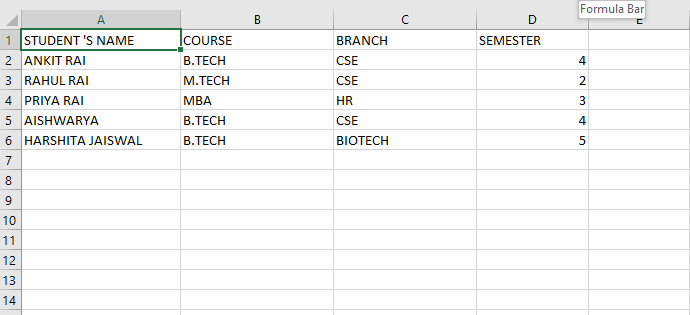Related Articles
Reading an excel file using Python openpyxl module
• Difficulty Level : Easy
• Last Updated : 13 Oct, 2020

`Openpyxl `is a Python library for reading and writing Excel (with extension xlsx/xlsm/xltx/xltm) files. The openpyxl module allows Python program to read and modify Excel files.

For example, users might have to go through thousands of rows and pick out a few handful of information to make small changes based on some criteria. Using Openpyxl module, these tasks can be done very efficiently and easily.

Use this command to install `openpyxl `module :

`sudo pip3 install openpyxl `

Input file :Code #1 : Program to print the particular cell value

 `# Python program to read an excel file`` ` `# import openpyxl module``import` `openpyxl`` ` `# Give the location of the file``path ``=` `"C:\\Users\\Admin\\Desktop\\demo.xlsx"`` ` `# To open the workbook ``# workbook object is created``wb_obj ``=` `openpyxl.load_workbook(path)`` ` `# Get workbook active sheet object``# from the active attribute``sheet_obj ``=` `wb_obj.active`` ` `# Cell objects also have a row, column, ``# and coordinate attributes that provide``# location information for the cell.`` ` `# Note: The first row or ``# column integer is 1, not 0.`` ` `# Cell object is created by using ``# sheet object's cell() method.``cell_obj ``=` `sheet_obj.cell(row ``=` `1``, column ``=` `1``)`` ` `# Print value of cell object ``# using the value attribute``print``(cell_obj.value)`

Output :

`STUDENT 'S NAME`

Code #2 : Determine total number of rows

 `# import openpyxl module``import` `openpyxl`` ` `# Give the location of the file``path ``=` `"C:\\Users\\Admin\\Desktop\\demo.xlsx"`` ` `# to open the workbook ``# workbook object is created``wb_obj ``=` `openpyxl.load_workbook(path)``sheet_obj ``=` `wb_obj.active`` ` `# print the total number of rows``print``(sheet_obj.max_row)`

Output :

`6`

Code #3 : Determine total number of columns

 `# importing openpyxl module``import` `openpyxl`` ` `# Give the location of the file``path ``=` `"C:\\Users\\Admin\\Desktop\\demo.xlsx"`` ` `# workbook object is created``wb_obj ``=` `openpyxl.load_workbook(path)`` ` `sheet_obj ``=` `wb_obj.active`` ` `# ptint total number of column ``print``(sheet_obj.max_column)`

Output :

`4`

Code #4 : Print all columns name

 `# importing openpyxl module``import` `openpyxl`` ` `# Give the location of the file``path ``=` `"C:\\Users\\Admin\\Desktop\\demo.xlsx"`` ` `# workbook object is created``wb_obj ``=` `openpyxl.load_workbook(path)`` ` `sheet_obj ``=` `wb_obj.active``max_col ``=` `sheet_obj.max_column`` ` `# Loop will print all columns name``for` `i ``in` `range``(``1``, max_col ``+` `1``):``    ``cell_obj ``=` `sheet_obj.cell(row ``=` `1``, column ``=` `i)``    ``print``(cell_obj.value)`

Output :

```STUDENT 'S NAME
COURSE
BRANCH
SEMESTER
```

Code #5 : Print first column value

 `# importing openpyxl module``import` `openpyxl`` ` `# Give the location of the file``path ``=` `"C:\\Users\\Admin\\Desktop\\demo.xlsx"`` ` `# workbook object is created``wb_obj ``=` `openpyxl.load_workbook(path)`` ` `sheet_obj ``=` `wb_obj.active``m_row ``=` `sheet_obj.max_row`` ` `# Loop will print all values``# of first column ``for` `i ``in` `range``(``1``, m_row ``+` `1``):``    ``cell_obj ``=` `sheet_obj.cell(row ``=` `i, column ``=` `1``)``    ``print``(cell_obj.value)`

Output :

```STUDENT 'S NAME
ANKIT RAI
RAHUL RAI
PRIYA RAI
AISHWARYA
HARSHITA JAISWAL
```

Code #6 : Print a particular row value

 `# importing openpyxl module``import` `openpyxl`` ` `# Give the location of the file``path ``=` `"C:\\Users\\Admin\\Desktop\\demo.xlsx"`` ` `# workbook object is created``wb_obj ``=` `openpyxl.load_workbook(path)`` ` `sheet_obj ``=` `wb_obj.active`` ` `max_col ``=` `sheet_obj.max_column`` ` `# Will print a particular row value``for` `i ``in` `range``(``1``, max_col ``+` `1``):``    ``cell_obj ``=` `sheet_obj.cell(row ``=` `2``, column ``=` `i)``    ``print``(cell_obj.value, end ``=` `" "``)`

Output :

`ANKIT RAI B.TECH CSE 4`

Attention geek! Strengthen your foundations with the Python Programming Foundation Course and learn the basics.

To begin with, your interview preparations Enhance your Data Structures concepts with the Python DS Course.

My Personal Notes arrow_drop_up